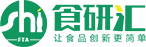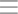0 92+

0 123+

0 117+

0 105+

0 131+

0 177+

0 105+

0 111+

0 207+

0 147+

4 173+

1 206+

1 237+

4 221+

1 159+

2 177+

1 139+

3 418+

1 209+

0 595+